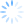CDXDistance2WP is the fastest distance calculation available in CDXZipStream. It calculates the straight-line ("as the crow flies") distance between two locations as defined by their latitude and longitude coordinates. This function is available in the Bing and higher versions of CDXZipStream.

CDXDistance2WP is an order of magnitude faster than the standard CDXDistance calculation and several order of magnitude faster than the Bing and Mappoint driving distance formulas.   There are no database calls as in CDXDistance to determine coordinates as they are already predefined.   And there are no delays waiting for Bing Maps or Mappoint to respond.

A question we often get asked is how to calculate determine the closest business or source locations to a long list of customer locations.   Attacking this type of problem with just the driving distance calculations can take many hours to run in a grid of 100K location pairs or more.  Once you geocode all the locations involved you can use the use the CDXDistance2WP function to determine straight line distance.  CDXDistance2WP is very fast and the analysis for a 100K grid should only take a few minutes. You can then determine the closest few locations for each client and then run a driving distance calculation for these top locations.  This dramatically cuts down on the calculation times.

We’ve developed an example template which shows how set up CDXDistance2WP on a simple five by five grid.  Just copy the formulas to new cells to expand it.  You can geocode locations using Bing or Mappoint or if only using zip code use the CDXZipCode formula to determine latitude and longitude.  Once calculated, set all the formulas to values and then sort the various columns/rows to determine the closest locations.  Setting the formulas to values avoids recalculation delays when sorting the lists.

We’ve also developed a “Geographic Analysis Template" that automates the calculation and sorting process described above and produces a custom output report that can be tailored by a specified radius.  Here is a video on how it works.

We have a variety of other articles on this blog describing the distance calculations available with CDXZipStream.  Here are some of the most popular ones.

https://blog.cdxtech.com/post/cdxzipstream-zip-code-distance-matrix-template